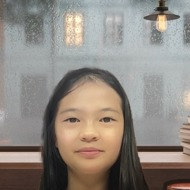# Charmaine

## Calculus/Differential equation/Middle&Elementary school mathematics.

Since I was younger, I had always enjoyed mathematics. From the age of 10, I started doing calculus. However, it wasn't just memorizing formulas, it was digging into the proofs and explanations of every theory. Therefore, teaching mathematics for me would be rather easy to explain. Furthermore, I wanted to express my love for mathematics by teaching others. A list of topics that I can teach are...
Calculus- from limits to derivatives, integrals, and Riemann sums, anything!
Differential equations- I can teach ODEs, but still, learn PDEs. But in ODEs, I can teach about Fourier transforms, solutions methods, boundary diff equations, and applications.
Middle/Elementary school math- Mathematics that is taught in middle school, which are fractions, algebraic manipulation, solving equations, equalities...

## 聯絡導師